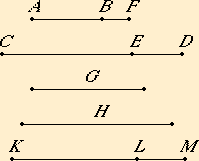# Proposition 114

If an area is contained by an apotome and the binomial straight line the terms of which are commensurable with the terms of the apotome and in the same ratio, then the side of the area is rational.

Let an area, the rectangle AB by CD, be contained by the apotome AB and the binomial straight line CD, and let CE be the greater term of the latter, let the terms CE and ED of the binomial straight line be commensurable with the terms AF and FB of the apotome and in the same ratio, and let the side of the rectangle AB by CD be G.

I say that G is rational.

Set out a rational straight line H, and to CD apply a rectangle equal to the square on H and producing KL as breadth. Then KL is an apotome.X.112

Let its terms be KM and ML commensurable with the terms CE and ED of the binomial straight line and in the same ratio.

But CE and ED are also commensurable with AF and FB and in the same ratio, therefore AF is to FB as KM is to ML.

V.19

Therefore, alternately, AF is to KM as BF is to LM. Therefore the remainder AB is to the remainder KL as AF is to KM.

But AF is commensurable with KM, therefore AB is also commensurable with KL.

And AB is to KL as the rectangle CD by AB is to the rectangle CD by KL, therefore the rectangle CD by AB is also commensurable with the rectangle CD by KL.

But the rectangle CD by KL equals the square on H, therefore the rectangle CD by AB is commensurable with the square on H.

But the square on G equals the rectangle CD by AB, therefore the square on G is commensurable with the square on H.

But the square on H is rational, therefore the square on G is also rational.

Therefore G is rational. And it is the side of the rectangle CD by AB.

Therefore, if an area is contained by an apotome and the binomial straight line the terms of which are commensurable with the terms of the apotome and in the same ratio, then the side of the area is rational.

Q.E.D.

# Corollary

And it is clear by this that it is possible for a rational area to be contained by irrational straight lines
.

## Guide

This proposition is not used in the rest of the Elements.